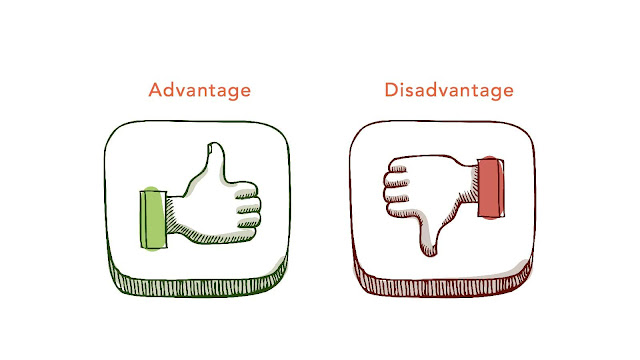# HSSlive: Plus One & Plus Two Notes & Solutions for Kerala State Board

## Monday, March 13, 2023Advantages And Disadvantages Of 2D Density And Hexbin Graph

## What is 2D Density And Hexbin Graph?

A 2D density plot and a hexbin plot are both types of data visualizations that are used to represent the distribution of data in two dimensions.

A 2D density plot, also known as a kernel density plot, uses color to represent the density of data points in a two-dimensional space. The plot is constructed by dividing the space into small cells and estimating the density of data points in each cell. The result is a smooth, continuous representation of the distribution of data.

A hexbin plot, on the other hand, uses hexagonal bins to represent the density of data points. The plot is constructed by dividing the space into hexagonal bins and counting the number of data points in each bin. The result is a plot that shows the density of data points in different regions of the two-dimensional space.

Both 2D density plots and hexbin plots are useful for visualizing the distribution of data in two dimensions, but they have different strengths and weaknesses. 2D density plots are better for showing the overall distribution of data and can reveal patterns in the data that may not be apparent with a hexbin plot. Hexbin plots are better for showing the local density of data and can reveal areas of high and low density more clearly than a 2D density plot.

## Advantages of 2D Density And Hexbin Graph

• Provide a smooth and continuous representation of the data distribution, which can reveal patterns that may not be visible with other types of plots.
• Useful for showing the overall distribution of data, including areas of high and low density.
• Can be used with large datasets and can accommodate different types of data.

• Useful for showing the local density of data and can reveal areas of high and low density more clearly than a 2D density plot.
• Can be used with large datasets and can accommodate different types of data.
• Can be more visually appealing than other types of density plots.

## Disadvantages of 2D Density And Hexbin Graph

• Can be difficult to interpret if the scale or color scheme is not well chosen.
• Can be sensitive to the choice of kernel function and bandwidth, which can affect the appearance of the plot.
• May not show the local density of data as well as a hexbin plot.

• Can be sensitive to the choice of bin size, which can affect the appearance of the plot.
• Can be difficult to interpret if the hexagons are too small or too large.
• May not show the overall distribution of data as well as a 2D density plot.

Overall, both 2D density plots and hexbin plots are useful for visualizing the distribution of data in two dimensions. The choice between the two will depend on the specific characteristics of the data and the research question at hand.

Share: